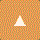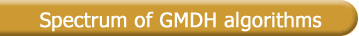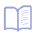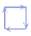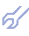Solution of practical problems and GMDH theory design lead to development of broad spectrum of software algorithms. Each of them corresponds to some definite conditions of it application . Algorithms mainly differ one from another by the models-candidates set generator arrangement for given basic function, by the way of models structure complexing and, at last, by the external criteria accepted. Algorithm choice depends on specifics of the problem, noise dispersion level, sufficiency of data sample, and if data sample contains only continuous data.

Most often criteria of accuracy, differential or informative type are used. The work of GMDH algorithms has a straightforward analogy with the work of gardener during selection of a new hybrid plant .

 GMDH algorithms Variables Parametric Non-parametric Continuous - Combinatorial (COMBI) - Multilayered Iterative (MIA) - GN - Objective System Analysis (OSA) - Harmonical - Two-level (ARIMAD) - Multiplicative-Additive (MAA) - Objective Computer Clusterization (OCC); - "Pointing Finger" (PF) clusterization algorithm; - Analogues Complexing (AC) Discrete or binary - Harmonical Rediscretization - Algorithm on the base of Multilayered Theory of Statistical Decisions (MTSD)

The basic parametric GMDH algorithms listed in table, have been developed for continuous variables. Among the parametric algorithms [1,9] the most known are:

- the basic Combinatorial (COMBI) algorithm. It is based on full or reduced sorting-out of gradually complicated models and evaluation of them by external criterion on separate part of data sample;

- Multilayered Iteration (MIA) algorithm use at each layer of sorting procedure the same partial description (iteration rule). It should be used when it is needed to handle a big number of variables;

- Objective System Analysis (OSA) algorithm. The key feature of it, is that it examine not single equations, but systems of algebraic or difference equations, obtained by implicit templates (without goal function). An advantage of the algorithm is that the number of regressors is increased consequently, the information embedded in the data sample is utilized better;

- Two-level (ARIMAD) algorithm for modeling of long-term cyclic (such as stock or weather) processes. There are used systems of polynomial or difference equations for identification of models on two time scales and then choice of the best pair of models by external criterion value. For this can be used any parametric algorithm from described above .

Less known are parametric algorithms, which apply an exhaustive search to difference, harmonic or harmonic-exponential functions, and the Multiplicative-Additive algorithm, in which tested polynomial models are obtained by taking the logarithm of the product of input variables [18,19].
The parametric GMDH algorithms have proved to be highly efficient in cases where one is to model objects with non-fuzzy characteristics, such as engineering objects. In cases, where modeling involves objects with fuzzy characteristics, it is more efficient to use the non-parametric GMDH algorithms, in which polynomial models are replaced by a data sample divided into intervals or clusters. Such type algorithms completely solve the problem of coefficients estimates bias elimination.

Non-parametric algorithms are exemplified by:

- Objective Computer Clusterization (OCC) algorithm that operates with pairs of closely spaced sample points . It finds physical clusterization that would as possible be the same on two subsamples;

- "Pointing Finger" (PF) algorithm for the search of physical clusterization. It is implemented by construction of two hierarchical clustering trees and estimation by the balance criterion ;

- Analogues Complexing (AC) algorithm, which use the set of analogues instead of models and clusterizations . It is recommended for the most fuzzy objects;

- Probabilistic algorithm, based on the Multilayered Theory of Statistical Decisions . It is recommended for recognition and forecasting of binary objects and for the veritability of input data control to avoid the possible experts errors in it.

Recent developments of the GMDH have led to neuronets with active neurons, which realise twice-multilayered structure: neurons are multilayered and they are connected into multilayered structure. This gives possibility to optimize the set of input variables at each layer, while the accuracy increases. The accuracy of forecasting, approximation or pattern recognition can be increased beyond the limits which are reached by neuronet with single neurons [9,10,34]. In this approach, which corresponds to the actions of human nervous system, the connections between several neurons are not fixed but change depending on the neurons themselves. Such active neurons are able during the learning self-organizing process to estimate which inputs are necessary to minimise the given objective function of neuron. This is possible on the condition that every neuron in its turn is multilayered unit, such as modeling GMDH algorithm. Neuronet with active neurons, is considered as a tool to increase AI problems accuracy and lead-time with the help of regression area extension for inaccurate, noisy data or small data samples.

The GMDH algorithms recently are applied in optimization to solve the problems of normative forecasting (after "what-if-then" scenario) and optimal control of multivariable ill-defined objects. Many ill-defined objects in macroeconomy, ecology, manufacturing etc. can be described accurately enough by static algebraic or by difference equations, which can be transformed into problems of linear programming by nomination of non-linear members by additional variables. GMDH algorithms are applied to evaluate deflections of output variables from their reference optimal values [7,21]. Examples of use of Simplified Linear Programming (SLP) algorithm should be used for expert computer advisers construction, normative forecasting and control optimization of averaged variables. An important example  gives the prediction of effects of experiments. The algorithm solves two problems: calculation of effects of a given nuclear experiment and calculation of parameters which are necessary to reach optimal effects. It means, that the realization of experiments can often be replaced by computer experiments.

As already noted, the GMDH algorithms have been developed for continuous variables. In practice, however the sample will often include variables discretized into a small number of levels or even binary values. To extend the GMDH algorithms to discretized or binary variables, the Harmonic Rediscretization algorithm has been developed .

The existence of a broad gamut of GMDH algorithms is traceable to the fact, that it is impossible to define the characteristics of the rest or controlled objects exactly in advance. Therefore, it can be good practice to try several GMDH algorithms one after another and to decide which one suits a given type of objects best.

All the questions, which arise during modeling process, are to be solved by the comparison of the criterion values: that variant is better, which leads to more deeper minimum of basic external criteria. In this way, the type of algorithm is chosen objectively, according to the value of the discriminating criterion.

Information about dispersion of noise level is very useful to chose the algorithm type. For small dispersion level we can use the learning networks of GMDH type, based on the ordinary regression analysis using internal criteria. For considerable noise level the GMDH algorithms with external criteria are recommended. And for high level of noise dispersion non-parametric algorithms of clusterization or analogues complexing should be applied .